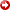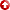--> --> Examples of Field Development Optimization Based on Joint Solution of Fluid Dynamics and Geomechanics on Common Model Grids presented by Dmitry Eydinov
[First Hit]# Examples of Field Development Optimization Based on Joint Solution of Fluid Dynamics and Geomechanics on Common Model Grids presented by Dmitry Eydinov

## Abstract

Objective and Scope In this work we consider onsolvingthe fluid flowequationstaking into account rock geomechanical deformations. The proposed solution is based on a joint system of fluid dynamicsequationsin porous media and elasticity theoryequationssolved on common 3D reservoir grid. Application examples of numerical model on real field cases will be provided. Methods, Procedures, Process The numerical model proposed in this work allows to run fine scale simulations of the reservoir fluid dynamics with full account for elastic rock deformations based on the elasticity theoryequationson the same grid used by the simulation model. The same discretization methods for both time and space are kept on both parts. The displacement vector representing deformations obtained from the solution of geomechanicalequationsis taken into account for the reservoir properties modeling at each time step. The pressure changes coming from the fluid dynamics, in turn, plays significant role in the stress conditions and rock compaction calculations. Thus, the joint model provides solution for reservoir simulation model with full account of the geomechanical effects within the common model grid. Results, Observations, Conclusions Analysis of geomechanical conditions of petroleum reservoirs is an essential part of the field development process. The geomechanical parameters, such as Young modulus and Poisson’s ratio can be obtained only from the well core samples. That requires additional experiments with cores, which are often omitted in the lab leaving the parameters very uncertain and the analysis very approximate. In the cross-hole space the parameters can only be assumed to be equal some average values. Using numerical solution of the fully coupled models with account of fluid dynamics and geomechanics, we recover the values of Poisson’s ratio and Young modulus from the history matching process and the uncertainty is reduced significantly. The hard data available from the studies on the core samples remains fixed, while the average values, parameter special distributions can be variated and adjusted so to match the historical production data. The values are homogeneous or heterogeneous depending on the reservoir complexity. In this work we demonstrate that by taking into account proper geomechanical effects in the reservoir, we significantly improve the quality of the built reservoir models and, thus, we improve the quality of the decisions on field development plan.